Next: Bibliography Up: Learning with Mixtures of Previous: Conclusions

Appendix A.

In this appendix we prove the following theorem from Section 6.2: Theorem Let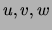be discrete variables such that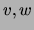do not co-occur with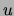(i.e.,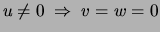in a given dataset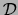). Let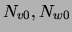be the number of data points for which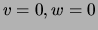respectively, and let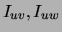be the respective empirical mutual information values based on the sample. Then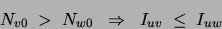with equality only ifis identically 0. Proof. We use the notation: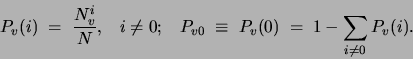These values represent the (empirical) probabilities of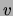taking value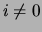and 0 respectively. Entropies will be denoted by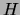. We aim to show that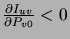. We first note a chain rule'' expression for the entropy of a discrete variable. In particular, the entropy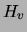of any multivalued discrete variablecan be decomposed in the following way: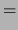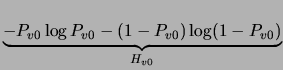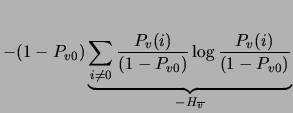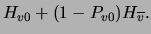(12)

Note moreover that the mutual information of two non-co-occurring variables is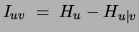. The second term, the conditional entropy ofgivenis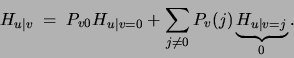We now expand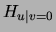using the decomposition in (12):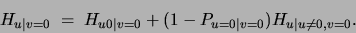Becauseandare never non-zero at the same time, all non-zero values ofare paired with zero values of. Consequently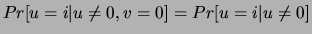and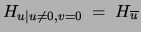. The term denoted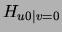is the entropy of a binary variable whose probability is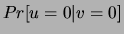. This probability equals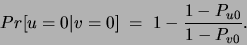Note that in order to obtain a non-negative probability in the above equation one needs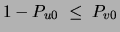, a condition that is always satisfied ifanddo not co-occur. Replacing the previous three equations in the formula of the mutual information, we get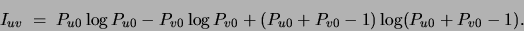This expression, remarkably, depends only on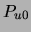and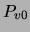. Taking its partial derivative with respect toyields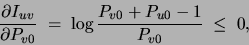a value that is always negative, independently of. This shows the mutual information increases monotonically with the occurrence frequency'' ofgiven by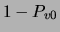. Note also that the above expression for the derivative is the same as the result obtained for binary variables in (11).Next: Bibliography Up: Learning with Mixtures of Previous: Conclusions
Journal of Machine Learning Research 2000-10-19